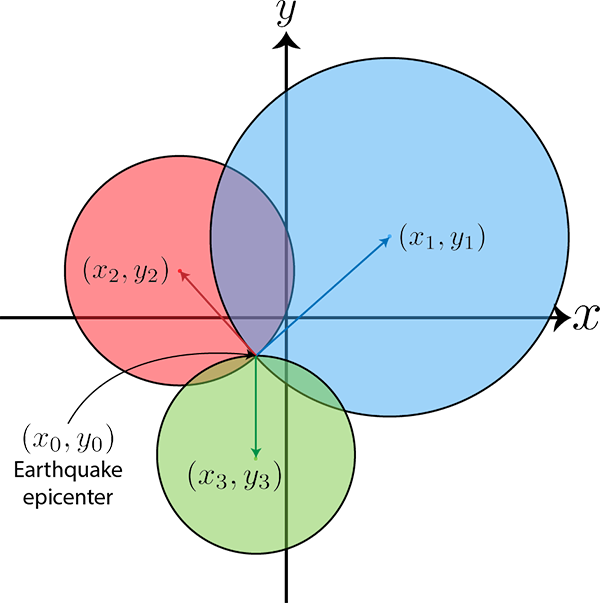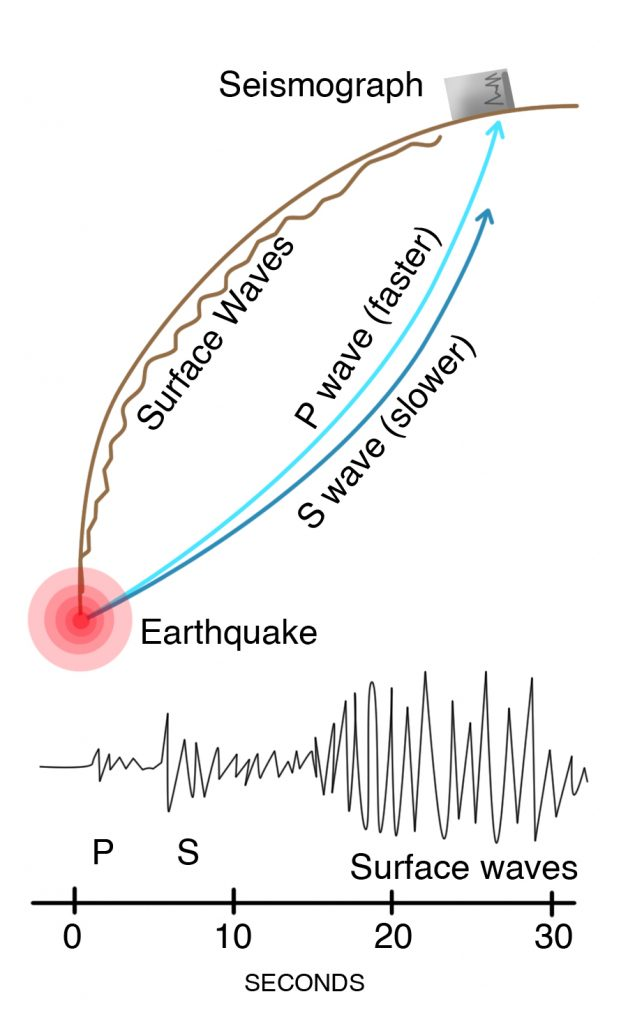Welcome to Project Lovelace! We're still in early development so there are still tons of bugs to find and improvements to make. If you have any suggestions, complaints, or comments please let us know on Discourse, Discord, or GitHub!

# Finding earthquake epicenters

## You will learn about: trilateration, equation of a circle, and systems of linear equations.

To figure out how far away an earthquake is you just need one seismograph but to pinpoint where the earthquake happened, it's epicenter, you need three seismographs in three different locations. Say an earthquake occurs at some unknown location $(x_0,y_0)$ on a 2D plane. It will emit seismic waves which travel through the Earth and are detected by seismograph stations. More than one type of wave is emitted but we'll just consider the faster compressional P-waves which travel at around $v = 6 \; \text{km/s}$ and arrive at the seismographs first.To give an idea of the problem, here's a schematic of the domain with the three seismographs at the centers of the colored circles. We can draw a circle around each seismograph whose radius is equal to the distance from the seismograph to the earthquake epicenter.The P-waves are faster and arrive at the seismograph before the shearing S-waves. They are both types of elastic body waves as they travel through the Earth with the P-waves being longitudinal while the S-waves being transverse. You can also think of the P and S as standing for primary and secondary. The surface waves are the slowest of the three and do the most damange as evidenced by the high ground motion registered on the seismograph. (Image credit: Living with Earthquakes in the Pacific Northwest, Yeats)

Given the position of three seismographs $(x_i,y_i)$ and arrival time of the seismic waves at each seismograph $t_i$ where $i=1,2,3$ as inputs, determine and return the earthquake's epicenter $(x_0,y_0)$.

Input: $(x_i,y_i)$ in kilometers where $-100 < x,y < 100$ and $t_i$ in seconds for $i=1,2,3$.

Output: The earthquake's epicenter $(x_0,y_0)$ in kilometers.

Example

Input x1: 8.382353226 Input y1: -58.003720814 Input t1: 12.860754193 Input x2: -13.590571819 Input y2: 73.976069096 Input t2: 22.847488548 Input x3: 77.291172370 Input y3: 7.508764456 Input t3: 5.767809783 Output x0: 79.06746127 Output y0: -27.05247781
 Difficulty earthquake_epicenter(x1, y1, t1, x2, y2, t2, x3, y3, t3)

You must be logged in to view your submissions.

### Notes

• The whole process of using points, distances, and circles to determine the location of another point (in our case the earthquake) is called trilateration and is how a GPS determines its location.
• Earthquakes usually happen below the surface so technically the epicenter is the location on the Earth's surface below which the earthquake occured while the earthquake's focus is where it actually happened inside the Earth.
• We're also assuming that the earthquake happens very close to the surface and that the seismographs are close to each other so the Earth's curvature is negligible. When the seismographs are far apart which is typical for earthquakes that happen below the ocean or when the earthquake happens deep below the ground, the Earth's spherical shape matters a lot.
• The actual velocity of the P-waves can vary quite a bit depending on the medium it's traveling through. We picked a typical value of 6 km/s which is roughly the velocity of a P-wave at the Earth's surface.

Let us know what you think about this problem! Was it too hard? Difficult to understand? Also feel free to discuss the problem, ask questions, and post cool stuff on Discourse. You should be able see a discussion thread below. Would be nice if you don't post solutions in there but if you do then please organize and document your code well so others can learn from it.